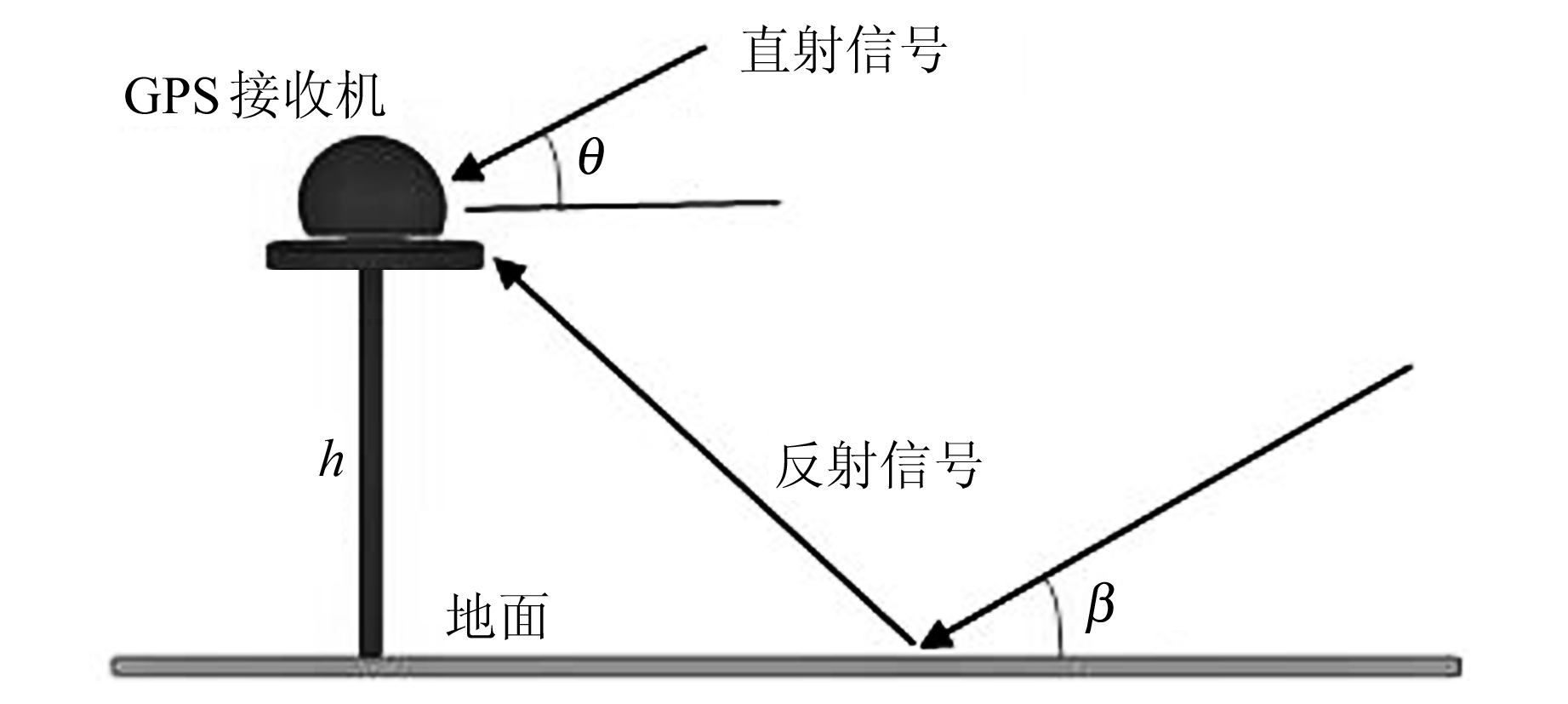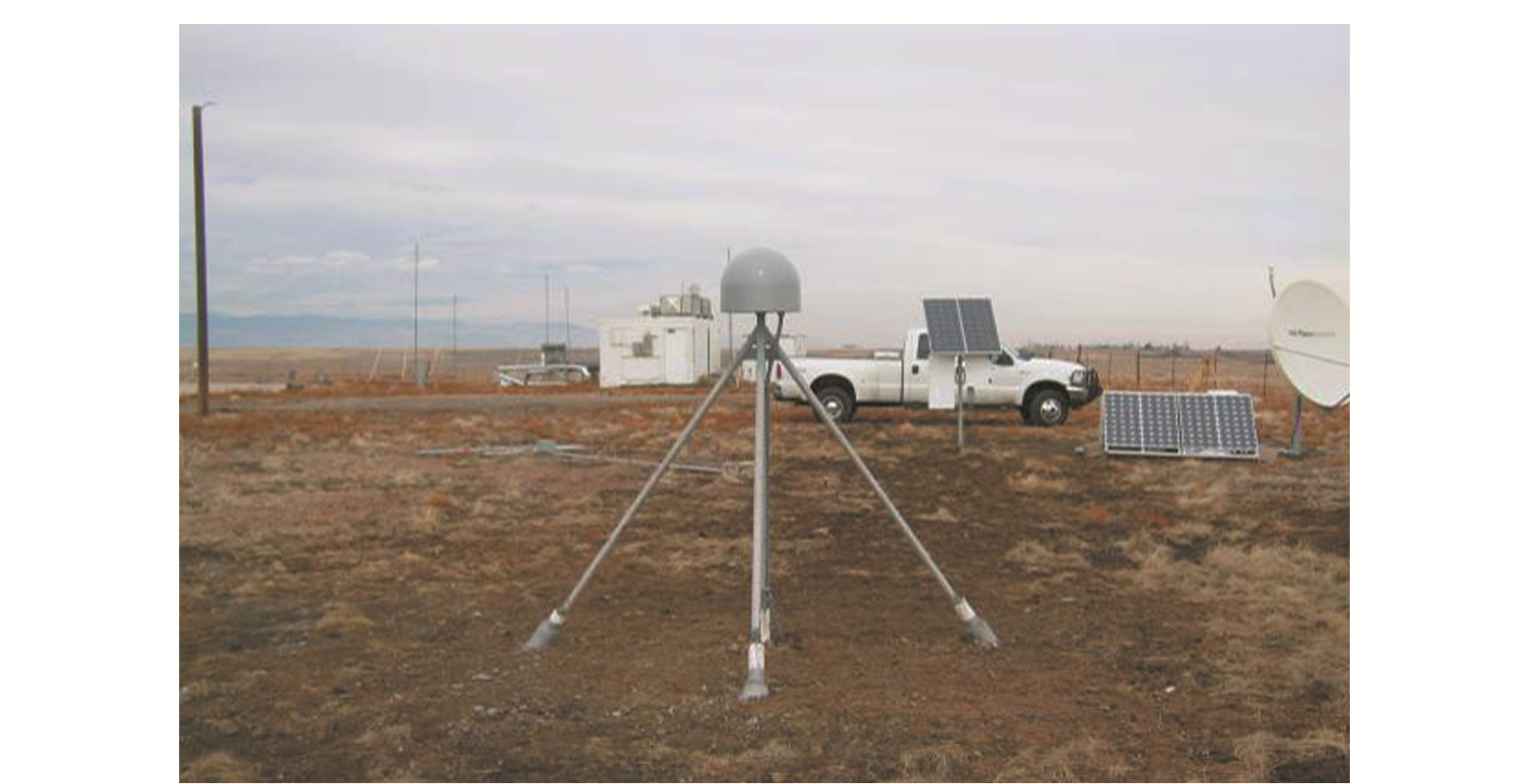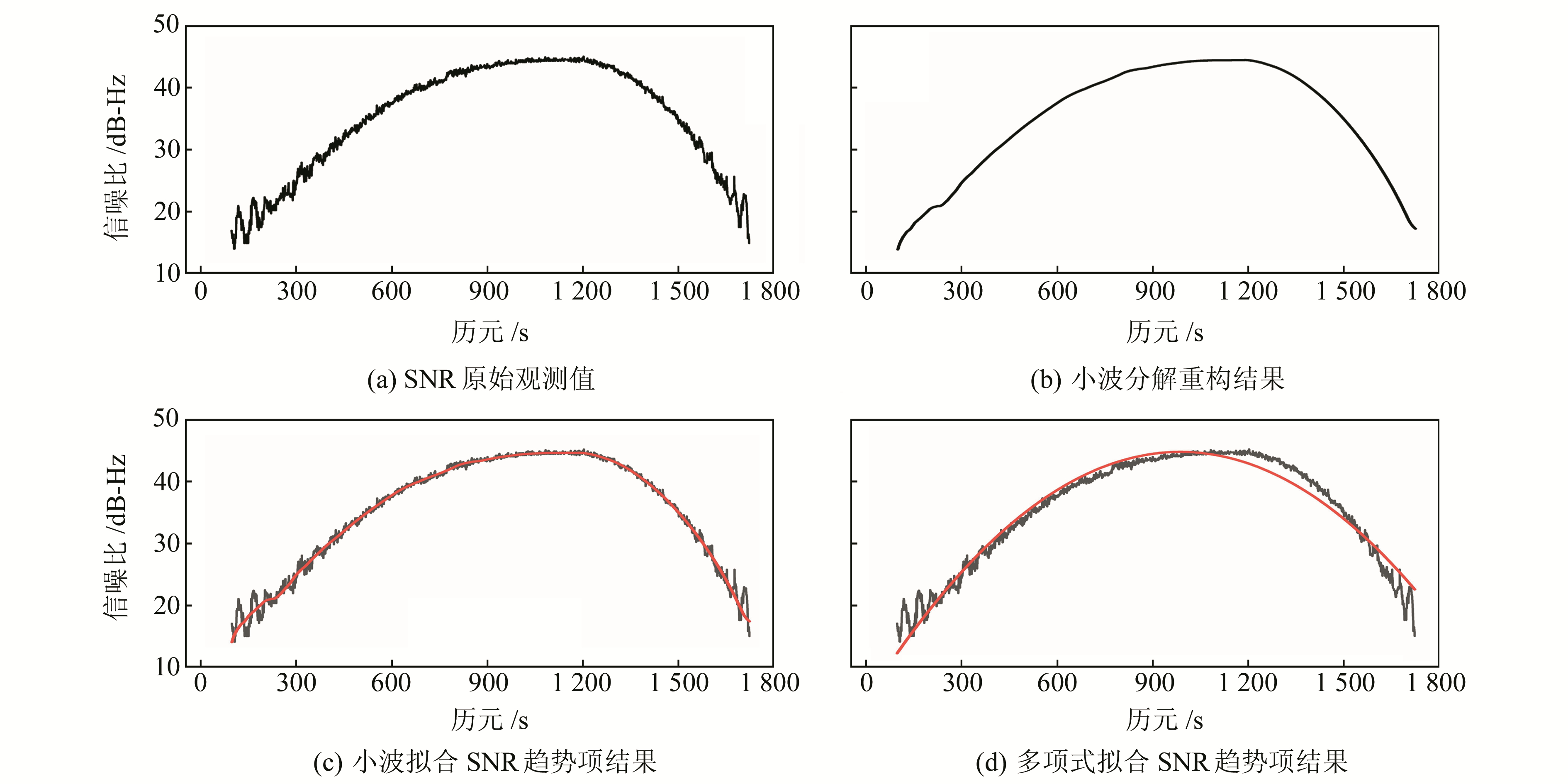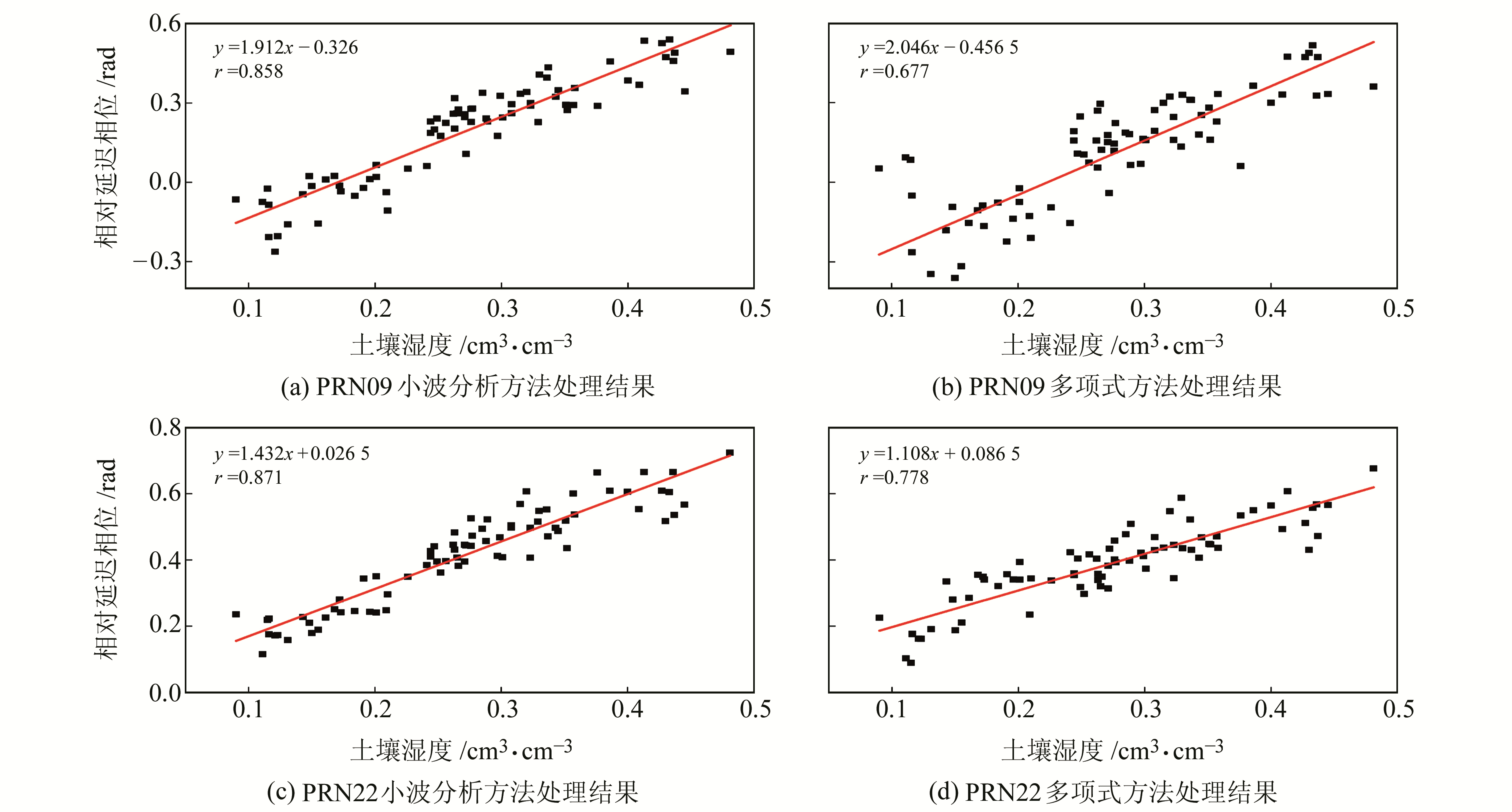﻿ 基于小波分析辅助GPS-IR反演土壤湿度研究文章快速检索 高级检索
 大地测量与地球动力学2020, Vol. 40Issue (1): 77-81  DOI: 10.14075/j.jgg.2020.01.015### 引用本文REN Chao, ZHANG Zhigang, LIANG Yueji, et al. Study on Inversion of Soil Moisture Using Wavelet Analysis Assisted GPS-IR[J]. Journal of Geodesy and Geodynamics, 2020, 40(1): 77-81.### Foundation support

National Natural Science Foundation of China,No.41901409,41461089; 2018 Guangxi Young and Middle-Aged Teachers’ Basic Ability Improvement Project,No.2018KY0247; Fund of Guangxi Key Laboratory of Spatial Information and Geomatics,No.16-380-25-22,15-140-07-34..

### 第一作者简介

REN Chao, PhD, professor, majors in GPS high precision data processing and its application，E-mail: renchao@glut.edu.cn..

### 文章历史

1. 桂林理工大学测绘地理信息学院，桂林市雁山街319号，541006;
2. 广西空间信息与测绘重点实验室，桂林市雁山街319号，541006

1 原理与方法 1.1 卫星信号反射原理

GPS-IR的核心观测值就是SNR，它是反映接收机天线信号质量的指标。SNR表达式为：

 $\text{SNR}={{\mathit{S}}_{\text{d}}}\left( 0 \right)+{{\mathit{S}}_{\text{m}}}\left( \varphi \right)\$ (1)图 1 地面多路径误差几何模型 Fig. 1 Ground multipath error geometry model

 $\frac{\text{d}\psi }{\text{d}t}=\frac{2\text{ }\!\!\pi\!\!\text{ }}{\lambda }2h~\frac{\text{d}(\text{sin}\theta )}{\text{d}t}$ (2)

 $\text{SN}{{\text{R}}_{\text{m}}}={{A}_{\text{m}}}\text{cos}\left( \text{ }\!\!~\!\!\text{ }\frac{4\text{ }\!\!\pi\!\!\text{ }h}{\lambda }\text{sin}\theta +\varphi \right)$ (3)

1.2 卫星反射信号分离原理

 \begin{align} & f\left( t \right)=\left[ x_{1}^{0}x_{2}^{0},\ldots ,x_{t}^{0} \right],~ \\ & \ \ \ \ \ t=1,2,3,\ldots ,I \\ \end{align}\ (4)

 ${{A}_{j}}\left[ f\left( t \right) \right]=\sum\limits_{k}{H\left( 2t-k \right){{A}_{j-1}}\left[ f\left( t \right) \right]}$ (5)
 ${{D}_{j}}\left[ f\left( t \right) \right]=\sum\limits_{k}{G\left( 2t-k \right){{A}_{j-1}}\left[ f\left( t \right) \right]}$ (6)

 \begin{align} & v{{A}_{j}}=2\left\{ \sum\limits_{k}{h\left( 2t-k \right){{A}_{j+1}}\left[ f\left( t \right) \right]+} \right. \\ & \ \ \ \ \ \ \left. \sum\limits_{k}{g\left( t-2k \right){{D}_{j+1}}\left[ f\left( t \right) \right]} \right\} \\ \end{align} (7)

2 实验分析图 2 P041测站 Fig. 2 P041 station图 3 PRN21号卫星的SNR观测值拟合趋势项 Fig. 3 SNR observation fitting trend term of PRN21 satellite表 1 两种方法的拟合精度统计 Tab. 1 Fitting accuracy statistics with two methods图 4 P041测站的相对相位延迟与土壤湿度的关系 Fig. 4 Relationship between relative delay phase and soil moisture of P041 station图 5 土壤湿度线性回归分析 Fig. 5 Linear regression analysis of soil moisture表 2 P041测站各卫星相位与土壤湿度的相关系数 Tab. 2 Correlation coefficient between satellite phase and soil moisture of P041 station
3 结语

  刘经南, 邵连军, 张训械, 等. GNSS-R研究进展及其关键技术[J]. 武汉大学学报:信息科学版, 2007(11): 955-960 (Liu Jingnan, Shao Lianjun, Zhang Xunxie, et al. Advances in GNSS-R Studies and Key Technologies[J]. Geomatics and Information Science of Wuhan University, 2007(11): 955-960) (0)  严颂华, 龚健雅, 张训械, 等. GNSS-R测量地表土壤湿度的地基实验[J]. 地球物理学报, 2011, 54(11): 2 735-2 744 (Yan Songhua, Gong Jianya, Zhang Xunxie, et al. Ground Based GNSS-R Observations for Soil Moisture[J]. Chinese Journal of Geophysics, 2011, 54(11): 2 735-2 744) (0)  Yu K G, Rizos C, Burrage D, et al. An Overview of GNSS Remote Sensing[J]. EURASIP Journal on Advances in Signal Processing, 2014, 134 (0)  Larson K M, Small E E, Gutmann E D, et al. Use of GPS Receivers as a Soil Moisture Network for Water Cycle Studies[J]. Geophysical Research Letters, 2008, 35(24): 851-854 (0)  Larson K M, Braun J J, Small L E E, et al. GPS Multipath and Its Relation to Near-Surface Soil Moisture Content[J]. IEEE Journal of Selected Topics in Applied Earth Observations and Remote Sensing, 2010, 3(1): 91-99 DOI:10.1109/JSTARS.2009.2033612 (0)  Bilich A, Larson K M. Mapping the GPS Multipath Environment Using the Signal to Noise Ratio(SNR)[J]. Radio Science, 2007(42): 3-13 (0)  Chew C, Small E E, Larson K M, et al. Effects of Near-Surface Soil Moisture on GPS SNR Data: Development of a Retrieval Algorithm for Soil Moisture[J]. IEEE Transactions on Geoscience and Remote Sensing, 2014, 52(1): 537-543 DOI:10.1109/TGRS.2013.2242332 (0)  敖敏思, 胡友健, 刘亚东, 等. GPS信噪比观测值的土壤湿度变化趋势反演[J]. 测绘科学技术学报, 2012, 29(2): 66-69 (Ao Minsi, Hu Youjian, Liu Yadong, et al. Inversion of Soil Moisture Fluctuation Based on Signal-to-Noise Ratio of Global Positioning System[J]. Journal of Geomatics Science and Technology, 2012, 29(2): 66-69) (0)  Wang X, Zhang Q, Zhang S. Water Levels Measured with SNR Using Wavelet Decomposition and Lomb-Scargle Periodogram[J]. GPS Solutions, 2018(1): 8 (0)  Ren C, Liang Y J, Lu X J, et al. Research on the Soil Moisture Sliding Estimation Method Using the LS-SVM Based on Multi-Satellite Fusion[J]. International Journal of Remote Sensing, 2018(10): 1-16 (0)  王祖林, 周荫清. 小波分析理论及其在雷达信号处理中的应用[J]. 北京航空航天大学学报, 1999(2): 10-13 (Wang Zulin, Zhou Yinqing. Wavelet Analysis Theory and Application in Radar Signal Processing[J]. Journal of Beijing University of Aeronautics and Astronautics, 1999(2): 10-13) (0)  丰秋林, 郑南山. 机器学习算法辅助的GPS信噪比观测值土壤湿度反演[J]. 测绘通报, 2018(7): 106-111 (Feng Qiulin, Zheng Nanshan. Retrieving Soil Moisture Using Signal-to-Noise Ratio of GPS Signal by Assisted Machine Learning Algorithm[J]. Bulletin of Surveying and Mapping, 2018(7): 106-111) (0)  罗甘, 梁月吉, 黄仪邦. 基于双树复小波的变形监测数据去噪分析[J]. 大地测量与地球动力学, 2018, 38(9): 958-963 (Luo Gan, Liang Yueji, Huang Yibang. Deformation Analysis Based on a Dual-Tree Complex Wavelet Transform Method[J]. Journal of Geodesy and Geodynamics, 2018, 38(9): 958-963) (0)  王旭, 王昶. 一种改进小波阈值去噪方法的研究[J]. 大地测量与地球动力学, 2017, 37(10): 1 038-1 041 (Wang Xu, Wang Chang. Improved Wavelet Threshold De-Noising Method Research[J]. Journal of Geodesy and Geodynamics, 2017, 37(10): 1 038-1 041) (0)
Study on Inversion of Soil Moisture Using Wavelet Analysis Assisted GPS-IR
REN Chao1,2ZHANG Zhigang1LIANG Yueji1,2     PAN Yalong1     HUANG Yibang1
1. College of Geomatics and Geoinformation, Guilin University of Technology, 319 Yanshan Street, Guilin 541006, China;
2. Guangxi Key Laboratory of Spatial Information and Geomatics, 319 Yanshan Street, Guilin 541006, China
Abstract: GPS-IR is a new remote sensing technology based on satellite reflection signals. The inversion of soil moisture can be achieved by using satellite reflection signals in SNR observations. Aiming at the problem of satellite reflection signal separation, we propose a satellite reflection signal separation method based on wavelet analysis. Experiments show that wavelet analysis can make corresponding changes to the signal-to-noise ratio observations of different satellites, effectively separating satellite direct signals and obtaining more accurate satellite reflection signals. Linear regression model can well represent relative delay phase and soil moisture. The correlation between the wavelet analysis and the low-order polynomial is more obvious. Therefore, the method can be used as a new method for separating satellite reflected signals.
Key words: GPS-IR; soil moisture; wavelet analysis; signal to noise ratio (SNR); satellite reflected signal